## Solution to 1986 Problem 20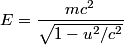\begin{align*}E = \frac{m c^2}{\sqrt{1 - u^2/c^2}}\end{align*}We are given that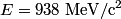$E = 938 \;\mathrm{MeV}\mathrm{/}\mathrm{c}^2$ and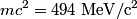$m c^2 = 494 \;\mathrm{MeV}\mathrm{/}\mathrm{c}^2$. Using the approximations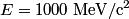$E = 1000 \;\mathrm{MeV}\mathrm{/}\mathrm{c}^2$ and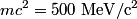$m c^2 = 500 \;\mathrm{MeV}\mathrm{/}\mathrm{c}^2$ in the above formula gives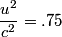\begin{align*}\frac{u^2}{c^2} = .75\end{align*}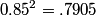$0.85^2 = .7905$ and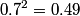$0.7^2 = 0.49$. Thus, answer (E) is correct.